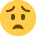Current updates regarding coronavirus (Covid-19) and the precautions AAT are taking will be continually updated on the below page.

We hope you are all safe and well and if you need us we will be here. 💚

# Stepped Costs

Hi

Does anyone know how to do stepped costs? I have the Osbourne text and nowhere does it tell you how to work out a stepped cost!

Question

Stepped cost increases with every 9000 Units.

Operating Statement 60,000

Sales Volume :27500 budgeted 26000 real units sold (I am flexing the budget purely on sales as there was no production figures)

What is the flexed budgeted Stepped cost amount if the 'actual' is 35000?

• Font Of All Knowledge Registered Posts: 2,453
• Font Of All Knowledge FMAAT, AAT Licensed Accountant Posts: 4,071
It's been years since I've done this but my logical approach would be:

actual production 35000
budget - increase per 9000
35000/9000 = 3.88

So there are 3 increments of 9000 i.e. 3 steps. Does that help?

Assuming I've remembered rightly and the flexed budget is the budgeted figures flexed or changed to reflect the actual production, i.e. what the budget would have been had the budget and actual production been the same quantity.

Your example doesn't say how much the cost of each 9000 units are. Lets say theres a budgeted base cost of £10,000 and +£5000 per 9000.

Then for 35,000 units the budgeted cost would be £10,000 + 4 x £5000 (or 3 x £5000 if you don't include the part-9000, I cant remember which is correct, but presumably 4x) so £30,000

I don't know whether the 60,000 is relevant. Costing was never my strong point.....

And I have no idea if this is right so hopefully someone else better than I will be along later on.

Monsoon

PS (6 hours isn't really enough time for a 'bump' - give it 24 hours for everyone to have a chance to have a look)
• Hi

Yes Monsoon is right, stepped cost is the cost per 9000 units. So you would divide the budgeted amount 27500 by 9000, to see how many lots of 9000 there were, this would be 4 as you've got into another lot of 9000 for the 500 left. You would then divide the cost of this expense (usually equipment hire) by 4 to find out how much each lot of 9000 cost. Once you know this you work out how many lots of 9000 you need for the actual amount sold (26000) which is 3 and multiply this by the cost per 9000, to arrive at your flexed figure.

Hope this is clear,

Leona
• Thanks

Hi

I cant belive it is so easy - wonder why Osbourne don't bother explaining it?

If you get this question wrong in the exam, you will drop 6 marks - the subtotal and total will be wrong as will the variances